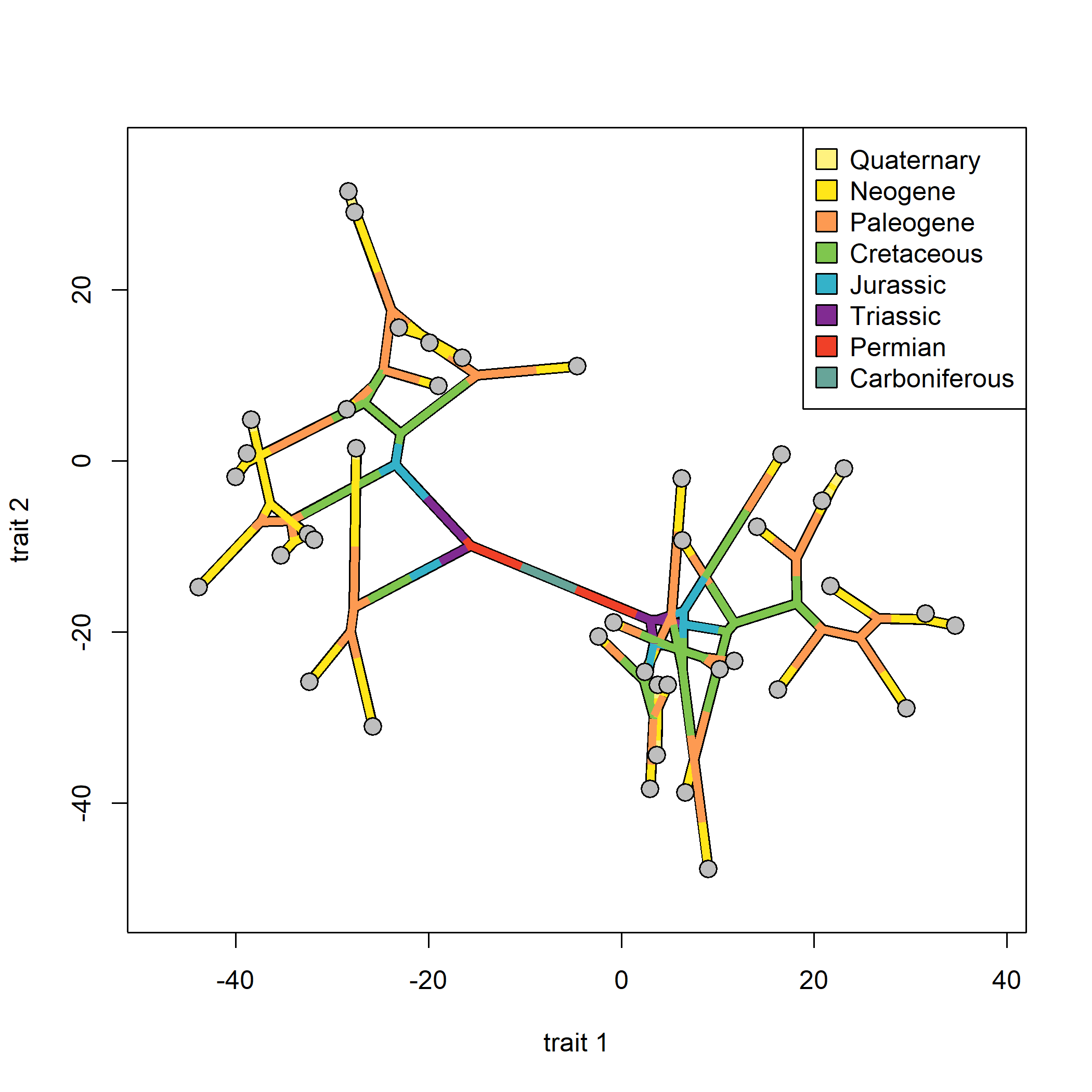## Monday, June 10, 2019

### Using `geo.palette( )` to map geological periods on a phylomorphospace plot

Yesterday I added a function to the namespace of phytools called `geo.palette` that returns the standard geological time scale color palette.

The following gives a demo on how this can be mapped onto a phylomorphospace:

``````## first compute total tree depth
h<-max(nodeHeights(tree))
## extract the temporal limits of your geological periods, but from
## the root of the tree forward
limits<-h-geo.palette()\$period[,"start"]
limits<-limits[limits>0]
limits<-c(0,sort(limits))
names(limits)<-rownames(geo.palette()\$period)[length(limits)]
limits
``````
``````## Carboniferous       Permian      Triassic      Jurassic    Cretaceous
##         0.000        21.100        67.830       118.700       175.000
##     Paleogene       Neogene    Quaternary
##       254.000       296.970       317.412
``````
``````## pull the colors from geo.palette( )
cols<-setNames(geo.palette()\$cols[length(limits):1],
names(limits))
## create our period-mapped tree using make.era.map
period.tree<-make.era.map(tree,limits)
## now plot our tree
## here I plot the phylomorphospace twice to get a black outline,
## but this is not necessary.
par(lend=1)
phylomorphospace(tree,X,lwd=7,node.size=c(0,0),
ftype="off",xlab="trait 1",ylab="trait 2")
phylomorphospace(period.tree,X,colors=cols,
points(X,pch=21,cex=1.5,bg="grey")
legend(x="topright",legend=names(cols)[length(cols):1],
pch=22,pt.bg=cols[length(cols):1],pt.cex=2)
``````My data for this exercise were simulated as follows:

``````set.seed(999)
tree<-pbtree(n=40,scale=320)
X<-fastBM(tree,nsim=2)
``````